# Fertilizer and Lime Calculations

Two basic pieces of information are needed to properly follow the fertilizer recommendation on a soil test.

1. How large is the area to be fertilized?

2. With the fertilizer that is available, how much needs to be applied to supply the recommended nutrients to this amount of area?

## How Large is the Area to be Fertilized?

The first step in applying fertilizer is to determine how many square feet of land is to be fertilized. This requires some geometry. At first, this may seem like a lot of work. However, you only have to do it once if you keep the information in a file.

Most of the following examples are for simple shapes. Choose the shape, or combination of shapes that most closely match the area to be fertilized to calculate the amount of land involved. Many land areas to be fertilized have a very irregular shape. In this case, you can use the offset method described in the final example.

## Shapes and Formulas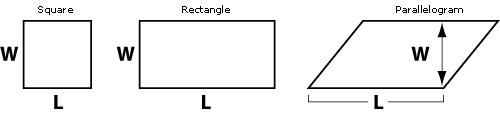These shapes are defined by the opposite sides being straight, parallel, and of equal length. The area of all 3 shapes is found by multiplying the length (L) times the width (W).

Formula

Area = L (length) x W (width)

Example

Area = L (length) x W (width)

L = 75 ft, W = 25 ft

• Area = 75 x 25

• =1875 ft2

## CircleThe area of a circle is found by multiplying the constant pi (p, or 3.14) times the square of the radius. The radius is ½ of the diameter.

Formula

Area = π x r2

π (pi) = 3.14

• r2 (radius squared) = r x r

Example

Area = π x r2

• r = 6 ft

Area = π x r2

• = 3.14 x (6 x 6)

• = 3.14 x 36

• = 113 ft2

## Triangle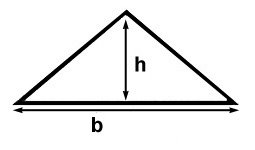The area of a triangle is found by multiplying the length of the base times the length of the height, then dividing this result by 2.

Formula

Area = (b x h) ÷ 2

• b = length of base

• h = length of height

Example

Area = (b x h) ÷ 2

• b = 10 ft, h = 5 ft

Area = (b x h) ÷ 2

• = (10 x 5) ÷ 2

• = 50 ÷ 2

• = 25 ft2

## Trapezoid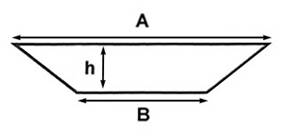The area of a trapezoid is found by first finding the average length of the parallel sides (A + B) ¸ 2, then multiplying the result times the height (h).

Formula

Area = [(A + B) ÷ 2] x h

Example

Area = [(A + B) ÷ 2] x h

• A = 20 ft, B + 10 ft, h = 5ft

Area = [{A + B) ÷ 2] x h

• = [(20 + 10) ÷ 2] x 5

• = [30 ÷ 2] x 5

• = 15 x 5

• = 75 ft2

## Oval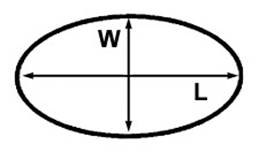The area of an oval is found by multiplying the width (W) times the length (L), then multiplying the result by 0.8

Formula

Area = (W x L) x 0.8

• W = width

• L = length

Example

Area = (W x L) x 0.8

• W = 10 ft , L = 20 ft

Area = (W x L) x 0.8

• = (10 x 20) x 0.8

• = 200 x 0.8

• = 160 ft2

## Fertilizing in an Area Around an Object or Tree

If you want to apply fertilizer an area around an object, such as a gazebo, separate landscaped area, or a conifer that has limbs touching the soil, you will need to make two calculations. Determine the area of the inner, unfertilized area using the appropriate formula, and then determine the size of the larger area that encompasses the inner object. Next, subtract the inner area from the outer area. The result will be the square footage of the area to be fertilized (the "doughnut shape" in this example).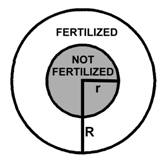Formula

Area to fertilize = Area of Outer Circle (π R2) - Area of Inner Circle (π r2)

Example

Area to fertilize = Area of Outer Circle (π R2) - Area of Inner Circle (π r2)

• R = 6 ft, r = 3 ft

Area to fertilize = Area of Outer Circle (π R2) - Area of Inner Circle (π r2)

• = [3.14 x (6 x 6)] - [3.14 x (3 x 3)]

• = [3.14 x 36] - [3.14 x 9]

• = 113 - 28

• = 85 ft2

## Compound Simple Shapes

Many landscape areas can be sub-divided into multiple, simple shapes. In these cases, use the formulas for the simple shapes and add the results for the total square footage. See the appropriate formula in other sections of this article.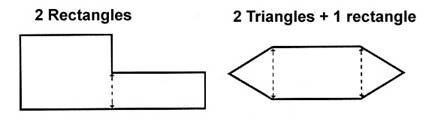## Odd Shapes

The method used for irregular shaped areas is called the "offset method". First measure the length of the longest axis of the area (line AB). This is called the length line. Next, divide the length line into equal sections, for example 10 ft. At each of these points, measure the distance across the area in a line perpendicular to the length line at each point (lines C through G). These lines are called offset lines. Finally, add the lengths of all offset lines and multiply the result times the distance that separates these lines (10 ft. in this example).Example

Length line (AB) = 60 ft., distance between offset lines is 10 ft apart

• Length of each offset line

C = 15 ft, D = 10 ft, E = 15 ft, F = 25 ft, G = 20 ft

Total length of offset lines = C + D + E + F + G

• = 15 + 10 + 15 + 25 + 20

• = 85 ft

Area to fertilize = Distance between offset lines x sum of the length of the offset lines

• = 10 ft x 85 ft

• = 850 ft2

## Other Formulas

Shape

Formula

Pentagon (5 equal sides)

(length of 1 side)2 x 1.7

Hexagon (6 equal sides)

(length of 1 side)2 x 2.6

Octagon (8 equal sides)

(length of 1 side)2 x 4.84